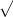# homework assignmentberett

 Question Part Points Submissions Used
Assume that x and y are both differentiable functions of t and find the required values of dy/dt and dx/dt.
y =x
(a) Find dy/dt, when x = 16, given that dx/dt = 4.
in2/min

(b)    r = 25 inches.
in2/min
decreasingFind the open intervals on which the function is increasing or decreasing. Use a graphing utility to verify your results. (Enter your answer using interval notation. If an answer does not exist, enter DNE.)
 increasing decreasing

STEP 2:Find the critical numbers. (Enter your answers as a comma-separated list.)
x =

STEP 3:Test the intervals to determine the open intervals on which the function is increasing or decreasing. (Enter your answer using interval notation.)
 increasing decreasing

Find the open intervals on which the function is increasing or decreasing. Use a graphing utility to verify your results. (Enter your answer using interval notation. If an answer does not exist, enter DNE.)
 increasing decreasing

Find the open intervals on which the function is increasing or decreasing. Use a graphing utility to verify your results. (Enter your answer using interval notation. If an answer does not exist, enter DNE.)
 increasing decreasing

Find the open intervals on which the function is increasing or decreasing. Use a graphing utility to verify your results. (Enter your answer using interval notation. If an answer does not exist, enter DNE.)
 increasing decreasing